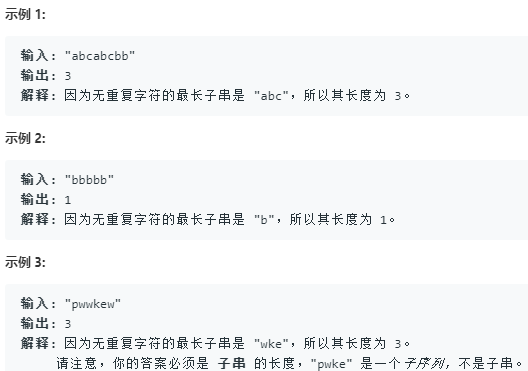# LeetCode - 3. 无重复字符的最长子串

### 3. 无重复字符的最长子串class Solution {
public:
int lengthOfLongestSubstring(string s) {
if (s.empty()) return 0;
int n = s.size(), i = 0, j = 0, res = 1;
unordered_map<char, int> m;
while (j < n) {
++m[s[j]];
if (s[j] >= 2) {
while (i <= j && m[s[j]] > 1) {
--m[s[i++]];
}
}
res = max(res, j - i + 1);
++j;
}
return res;
}
};©️2019 CSDN 皮肤主题: 创作都市 设计师: CSDN官方博客